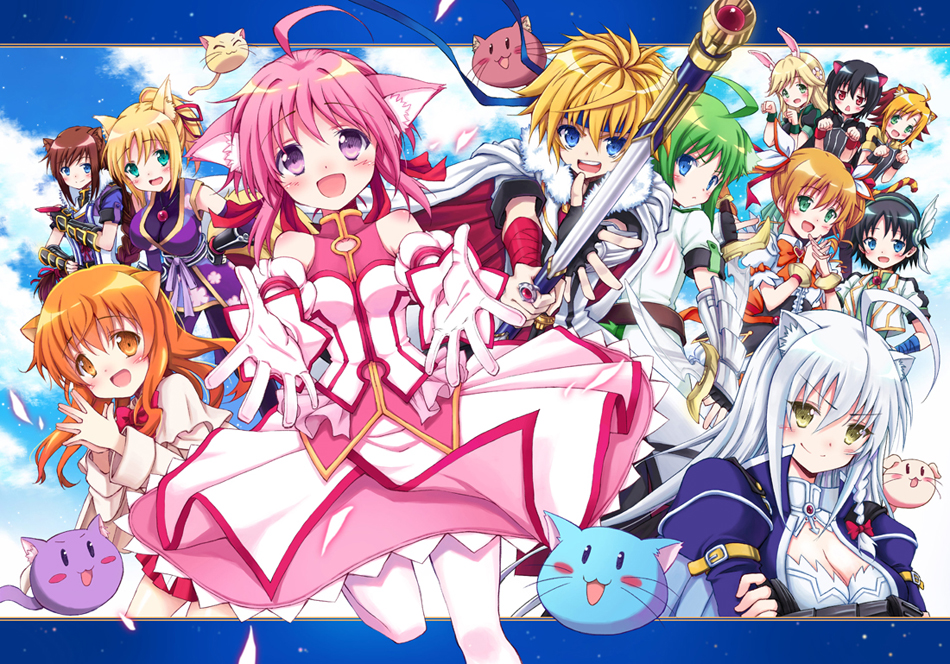# Dog Days Season 3 Announcedちょっとまってください！！！Stop everything folks cause you've just been drafted into war... again!

Enter the realm of Flonyard for a battle to the 'death'. Are you brave enough to enter the heat of the battle? Did I mention that this war is made of sport events? Yep! For those who haven't watched Dog Days yet go check it out if you dare! (I only watched it because Mizuki Nana was voicing characters! Shows how much of a fanboy I really am...)

The illustrator of Dog Days' manga has announced that a third season of the series is being made. No date has been announced yet, so an assumption (on a lot of people's part) for 2013 has been made!

Anyone down for more Cinque, Becky, and Nanami going back to Flonyard action? Me is more of a so-so. Just watch it because Mizuki Nana voices both Nanami and Ricotto! Gah!! It would have been better if they made the Flonyard crew come to Modern Day Japan, would have been nice and something new in the series!

Anyway, I'm patiently waiting for this...• Blogger Comments
• Facebook Comments

#### 15 comments:

1.I hope it comes out soon!! i loved the first two seasons and i can't wait to see what happens next!! so lovey-dovey!! :) :d

2.3."It would have been better if they made the Flonyard crew come to Modern Day Japan"
Me too!My hope is in season 3!

4.f*** ya seson 3!!!!!!!!!!!!!!!! =)) =)) =)) =)) =)) =)) =)) =)) =)) =)) =)) =)) =)) =)) =)) =)) =)) =)) =)) =)) =)) =)) =)) =)) =)) =)) =)) =)) =)) =)) =)) =)) =)) =)) =)) =)) =)) =)) =)) =)) =)) =)) =)) =)) =)) =)) =)) =)) =)) =)) =)) =)) =)) =)) =)) =)) =)) =)) =)) =)) =)) =)) =)) =)) =)) =)) =)) =)) =)) =)) =)) =)) =)) =)) =)) =)) =)) =)) =)) =)) =)) =)) =)) =)) =)) =)) =)) =)) =)) =)) =)) =)) =)) =)) =)) =)) =)) =)) =)) =)) =)) =)) =)) =)) =)) =)) =)) =)) =)) =)) =)) =)) =)) =)) =)) =)) =)) =)) =)) =)) =)) =)) =)) =)) =)) =)) =)) =)) =)) =)) =)) =)) =)) =)) =)) =)) =)) =)) =)) =)) =)) =)) =)) =)) =)) =)) =)) =)) =)) =)) =)) =)) =)) =)) =)) =)) =)) =)) =)) =)) =)) =)) =)) =)) =)) =)) =)) =)) =)) =)) =)) =)) =)) =)) =)) =)) =)) =)) =)) =)) =)) =)) =)) =)) =)) =)) =)) =)) =)) =)) =)) =)) =)) =)) =)) =)) =)) =)) =)) =)) =)) =)) =)) =)) =)) =)) =)) =)) =)) =)) =)) =)) =)) =)) =)) =)) =)) =)) =)) =)) =)) =)) =)) =)) =)) =)) =)) =)) =)) =)) =)) =)) =)) =)) =)) =)) =)) =)) =)) =))

1.OMG ima so happy i could die...zombieness

2.omg so happy

5.Hope there be season three because it is fun to watch it and really funny and awesome shows! :)

6.I can`t wait untill season 3 beacause I watched 2 seasons of dog days two times! This story is really funny and exciting. I haven`t ever wathced so cool anime :)). BTW I recommend you guys as cool anime. It`s
"Mondaiji-tachi ga Isekai kara Kuru Sou Desu yo?". Love this too.

7.i absolutely CANNOT wait for season 3 to come out!
:)

8.Cant wait to see Shinku and Millhoire Moments <3

9.Just thought of something! What if Nanami's two friends came along, so there could be 5 heroes! 5 way war would be amazing! :)

10.I just thought of something! What if Nanami's two friends came to Flonyard! 5 heroes, meaning two more countries can enter the fray! Would be really cool! Also if they could make more than 13 episodes *knocks on head*.

11.Can't wait for season 3, just finished 1 and 2. I hope they go back towards season 1 tone wise, I wasn't much of a fan of the direction that season 2 went, emphasizing harem-fan service a tad too much, losing the aspect of discovery and hard training, back-seating some of the more enjoyable characters from season 1, and loosing a sense of urgency from a central plot line that season 1 offered through (Season 1 spoiler alert) Leo's unconventional actions to prevent impending death for the main characters (spoiler end). Anyway, still excited for it!

12.when is season 3 coming our? :-?

13.when does it come out?

Top